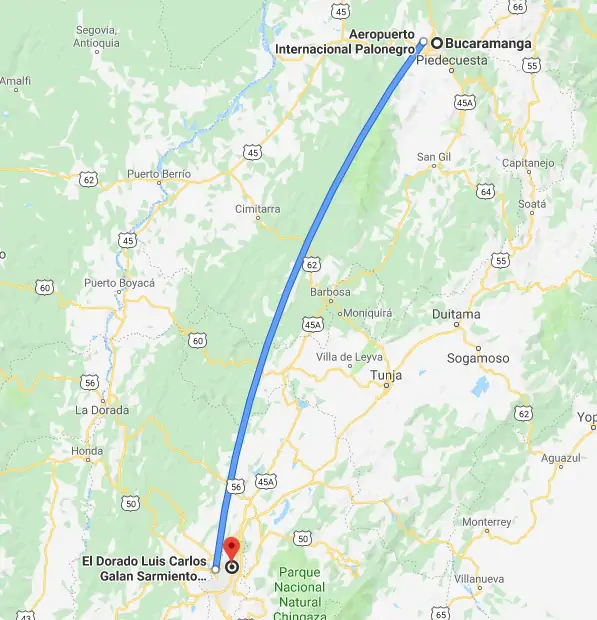How to calculate the distance between 2 markers (coordinates) in Google Maps with JavaScript

One of the most recurrent needs of developers when working with Google Maps, is how to obtain the distance between 2 different points on the map, usually defined by Markers or just by plain coordinates, for example given the following code:

var map, markerA, markerB;

// Callback executed when Google Maps script has been loaded
function initMap() {
// 1. Initialize map
map = new google.maps.Map(document.getElementById('map'), {
zoom: 10,
center: {
lat: 6.066385702972249,
lng: -74.07493328924413
}
});

// 2. Put first marker in Bucaramanga
markerA = new google.maps.Marker({
position: {
lat: 7.099473939079819,
lng: -73.10677064354888
},
map: map,
icon: {
},
title: "Marker A"
});

// 3. Put second marker in Bogota
markerB = new google.maps.Marker({
position: {
lat: 4.710993389138328,
lng: -74.07209873199463
},
map: map,
icon: {
},
title: "Marker B"
});
}

This will place the 2 markers that appear on the picture of this article. According to Google, we can know that the flight distance from Bucaramanga to Bogota is about 280 Kilometers:So we will theorically expect an approximated value from our methods to obtain the distance between the 2 coordinates. In this article, we will show you 2 ways to obtain the distance between 2 markers or coordinates with JavaScript in Google Maps.

A. Using Google Geometry Module

By default, Google provides a custom way to obtain the distance between 2 points on the map using the Geometry library. You need to include this library by appending the &libraries get parameter to the request URL of the Google Maps JS API:

<script async defer src="https://maps.googleapis.com/maps/api/js?key=YOUR_KEY&callback=initMap&libraries=geometry"></script>

Once you are sure that have loaded this library, you can now obtain the distance between 2 coordinates (LatLng object) from Google Maps through the google.maps.geometry.spherical.computeDistanceBetween method. For example, if you want to obtain the distance between 2 markers, you may simply run the following instruction:

// Obtain the distance in meters by the computeDistanceBetween method
// From the Google Maps extension
var distanceInMeters = google.maps.geometry.spherical.computeDistanceBetween(
markerA.getPosition(),
markerB.getPosition()
);

// Outputs: Distance in Meters:  286562.7470149898
console.log("Distance in Meters: ", distanceInMeters);

// Outputs: Distance in Kilometers:  286.5627470149898
console.log("Distance in Kilometers: ", (distanceInMeters * 0.001));

As you can see, the computeDistanceBetween method expects as first and second argument a LatLng object. It will compute the distance from those 2 parameters and return the value in meters. Is up to you to change the units mathematically to Miles or Kilometers. Alternatively if you are not using markers, you may provide the mentioned LatLng object as arguments with the direct coordinates:

// Obtain the distance in meters by the computeDistanceBetween method
// From the Google Maps extension using plain coordinates
var distanceInMeters = google.maps.geometry.spherical.computeDistanceBetween(
lat: 7.099473939079819,
lng: -73.10677064354888
}),
lat: 4.710993389138328,
lng: -74.07209873199463
})
);

// Outputs: Distance in Meters:  286562.7470149898
console.log("Distance in Meters: ", distanceInMeters);

// Outputs: Distance in Kilometers:  286.5627470149898
console.log("Distance in Kilometers: ", (distanceInMeters * 0.001));

B. Using the Haversine formula

If instead of relying on Google Maps, you can calculate the same distance based on the Haversine Formula, an important equation used in navigation, this provides great-circle distances between two points on a sphere from their longitudes and latitudes. It is a special case of a more general formula in spherical trigonometry, the law of haversines, relating the sides and angles of spherical "triangles".

To start, include the 2 following functions in your code:

/**
* Converts degrees to radians.
*
* @param degrees Number of degrees.
*/
return degrees * Math.PI / 180;
}

/**
* Returns the distance between 2 points of coordinates in Google Maps
*
* @see https://stackoverflow.com/a/1502821/4241030
* @param lat1 Latitude of the point A
* @param lng1 Longitude of the point A
* @param lat2 Latitude of the point B
* @param lng2 Longitude of the point B
*/
function getDistanceBetweenPoints(lat1, lng1, lat2, lng2){
// The radius of the planet earth in meters
let R = 6378137;
let dLat = degreesToRadians(lat2 - lat1);
let dLong = degreesToRadians(lng2 - lng1);
let a = Math.sin(dLat / 2)
*
Math.sin(dLat / 2)
+
*
*
Math.sin(dLong / 2)
*
Math.sin(dLong / 2);

let c = 2 * Math.atan2(Math.sqrt(a), Math.sqrt(1 - a));
let distance = R * c;

return distance;
}

The first function allows you to convert a degree into radians, necessary in the function of interest, the getDistanceBetweenPoints method. In this case, with a slightly modification from the original snippet of RosettaCode. The function receives four arguments that correspond respectively to the latitude and longitude values of both points. This solution doesn't rely on Google Maps so it can be used in other projects as well:

// Obtain the distance in meters using the haversine formula
var distanceInMeters = getDistanceBetweenPoints(
// LatA
7.099473939079819,
// LongA
-73.10677064354888,
// LatB
4.710993389138328,
// LongB
-74.07209873199463
);

// Outputs: Distance in Meters:  286476.96153465303
console.log("Distance in Meters: ", distanceInMeters);

// Outputs: Distance in Kilometers:  286.476961534653
console.log("Distance in Kilometers: ", (distanceInMeters * 0.001));

Happy coding !